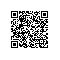# 我的入门学习

HashMap原理分析

public class HashMap extends AbstractMap

implements Map, Cloneable, Serializable {

//默认初始化容量
static final int DEFAULT_INITIAL_CAPACITY = 1 << 4;

//最大容量
static final int MAXIMUM_CAPACITY = 1 << 30;

//默认负载因子
static final float DEFAULT_LOAD_FACTOR = 0.75f;

//链表转红黑树阈值
static final int TREEIFY_THRESHOLD = 8;

//取消阈值
static final int UNTREEIFY_THRESHOLD = 6;

//最小树容量
static final int MIN_TREEIFY_CAPACITY = 64;
}

public HashMap() {

this.loadFactor = DEFAULT_LOAD_FACTOR; 

}

public HashMap(int initialCapacity) {

this(initialCapacity, DEFAULT_LOAD_FACTOR);

}

public HashMap(int initialCapacity, float loadFactor) {

if (initialCapacity < 0)
throw new IllegalArgumentException("Illegal initial capacity: " +
initialCapacity);
if (initialCapacity > MAXIMUM_CAPACITY)
initialCapacity = MAXIMUM_CAPACITY;
throw new IllegalArgumentException("Illegal load factor: " +
this.threshold = tableSizeFor(initialCapacity);

}

static final int tableSizeFor(int cap) {

int n = cap - 1;
n |= n >>> 1;
n |= n >>> 2;
n |= n >>> 4;
n |= n >>> 8;
n |= n >>> 16;
return (n < 0) ? 1 : (n >= MAXIMUM_CAPACITY) ? MAXIMUM_CAPACITY : n + 1;

}

0000 0000 0000 0000 0000 0000 0000 0101 cap = 5

0000 0000 0000 0000 0000 0000 0000 0100 n = cap - 1

0000 0000 0000 0000 0000 0000 0000 0010 n >>> 1

0000 0000 0000 0000 0000 0000 0000 0110 n |= n >>> 1

0000 0000 0000 0000 0000 0000 0000 0001 n >>> 2

0000 0000 0000 0000 0000 0000 0000 0111 n |= n >>> 2

0000 0000 0000 0000 0000 0000 0000 0000 n >>> 4

0000 0000 0000 0000 0000 0000 0000 0111 n |= n >>> 4

0000 0000 0000 0000 0000 0000 0000 0000 n >>> 8

0000 0000 0000 0000 0000 0000 0000 0111 n |= n >>> 8

0000 0000 0000 0000 0000 0000 0000 0000 n >>> 16

0000 0000 0000 0000 0000 0000 0000 0111 n |= n >>> 16

public V put(K key, V value) {

return putVal(hash(key), key, value, false, true);

}

static final int hash(Object key) {

int h;
return (key == null) ? 0 : (h = key.hashCode()) ^ (h >>> 16);

}

final V putVal(int hash, K key, V value, boolean onlyIfAbsent,

           boolean evict) {
Node[] tab; Node p; int n, i;

// 步骤【1】：tab为空扩容
if ((tab = table) == null || (n = tab.length) == 0)
n = (tab = resize()).length;

// 步骤【2】：计算index，并对null做处理
if ((p = tab[i = (n - 1) & hash]) == null)
tab[i] = newNode(hash, key, value, null);
else {
Node e; K k;

// 步骤【3】：键存在，直接覆盖值
if (p.hash == hash &&
((k = p.key) == key || (key != null && key.equals(k))))
e = p;

// 步骤【4】：若为红黑树
else if (p instanceof TreeNode)
e = ((TreeNode)p).putTreeVal(this, tab, hash, key, value);
else {

// 步骤【5】：若为链表
for (int binCount = 0; ; ++binCount) {
if ((e = p.next) == null) {
p.next = newNode(hash, key, value, null);

//若链表长度大于8则转换为红黑树进行处理
if (binCount >= TREEIFY_THRESHOLD - 1)
treeifyBin(tab, hash);
break;
}
if (e.hash == hash &&
((k = e.key) == key || (key != null && key.equals(k))))
break;
p = e;
}
}
if (e != null) {
V oldValue = e.value;
if (!onlyIfAbsent || oldValue == null)
e.value = value;
afterNodeAccess(e);
return oldValue;
}
}
++modCount;

// 步骤【6】：超过最大容量进行扩容
if (++size > threshold)
resize();
afterNodeInsertion(evict);
return null;

}

if ((p = tab[i = (n - 1) & hash]) == null)

    tab[i] = newNode(hash, key, value, null);

0000 0000 0000 0000 0000 0000 0001 1111 hash = 31
0000 0000 0000 0000 0000 0000 0000 1111 n - 1
0000 0000 0000 0000 0000 0000 0000 1111 => 15

0000 0000 0000 0000 0000 0000 0011 1111 hash = 63
0000 0000 0000 0000 0000 0000 0000 1111 n - 1
0000 0000 0000 0000 0000 0000 0000 1111 => 15

0000 0000 0000 0000 0000 0000 0111 1111 hash = 95
0000 0000 0000 0000 0000 0000 0000 1111 n - 1
0000 0000 0000 0000 0000 0000 0000 1111 => 15

final Node[] resize() {

Node[] oldTab = table;
int oldCap = (oldTab == null) ? 0 : oldTab.length;
int oldThr = threshold;
int newCap, newThr = 0;
if (oldCap > 0) {
if (oldCap >= MAXIMUM_CAPACITY) {
threshold = Integer.MAX_VALUE;
return oldTab;
}
else if ((newCap = oldCap << 1) < MAXIMUM_CAPACITY &&
oldCap >= DEFAULT_INITIAL_CAPACITY)
newThr = oldThr << 1;
}
else if (oldThr > 0)
newCap = oldThr;
else {
newCap = DEFAULT_INITIAL_CAPACITY;
}
if (newThr == 0) {
float ft = (float)newCap * loadFactor;
newThr = (newCap < MAXIMUM_CAPACITY && ft < (float)MAXIMUM_CAPACITY ?
(int)ft : Integer.MAX_VALUE);
}
threshold = newThr;
......

}

int index = (n - 1) & hash;

int index = (hash & 0x7FFFFFFF) % tab.length;